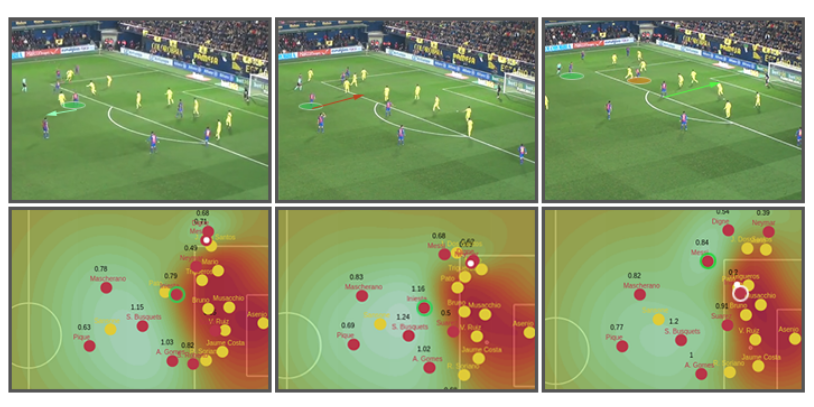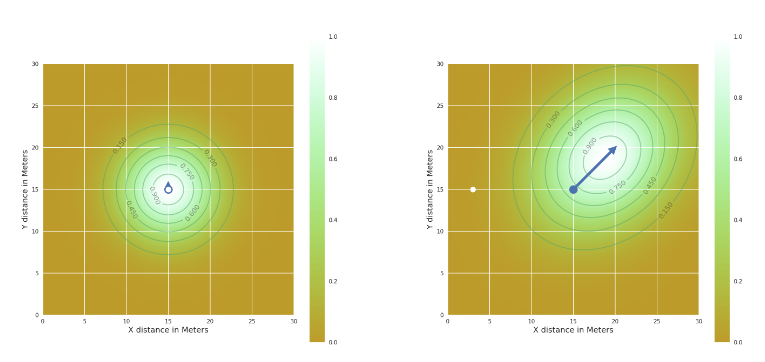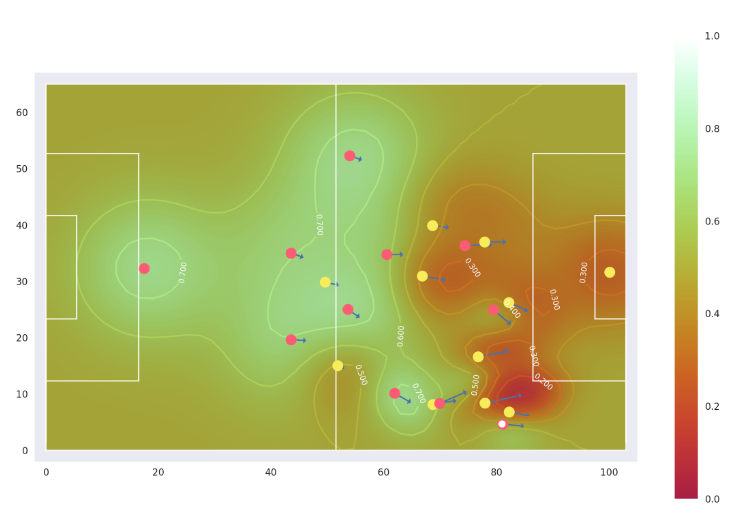2019/04/10 10:10

# 前言

1. 该篇分享来源于NFL竞赛官方的R语言版本，我做的主要是翻译为Python版本；
2. 分享中用到的技巧、构建的特征、展示数据的方式都可以应用到其他领域，比如篮球、足球、LOL、双人羽毛球等等，只要是团队竞技，都可以从中获益；
3. 分享基于kaggle上的NFL大数据碗，也就是基于橄榄球；
4. 泰森多边形的概念最好可以去了解一下，可以不用纠结于公式，看看它对一些实际问题的抽象建模表示即可；

## 分享目录

1. 使用matplotlib对比赛实况进行绘制，直观理解某一时刻下的球场状态；
2. 使用泰森多边形可视化各个球员的控制区域，借以理解、量化当前的形势；
3. 结合球员们的当前位置、速度、加速度、方向等信息绘制行进路线图，可视化在N秒后的状态；
4. 分享一篇去年关于足球球员控制区域热图相关的论文中的信息，这部分没有在项目里，大家感兴趣可以看看这里

## 竞赛链接

https://www.kaggle.com/c/nfl-big-data-bowl-2020

## 项目链接，该项目代码已经public，大家可以copy下来直接运行

https://www.kaggle.com/holoong9291/nfl-tracking-wrangling-voronoi-and-sonars-python

## github仓库链接，更多做的过程中的一些思考、问题等可以在我的github中看到

https://github.com/NemoHoHaloAi/Competition/tree/master/kaggle/Top61%25-0.01404-zzz-NFL-Big-Data-Bowl

## 一些橄榄球相关的基本概念

• 美式足球：进攻方目的是通过跑动、传球等尽快抵达对方半场，也就是达阵，而防守方的目的则是相反，尽全力去阻止对方的前进以及尽可能断球；
• 球场长120码(109.728米），宽53码（48.768米），周长是361.992米；
• 球员：双方场上共22人，进攻方11人，防守方11人，进攻方持球；
• 进攻机会：进攻方共有四次机会，需要推进至少十码；
• 进攻方：进攻方的职责是通过四次机会，尽可能的向前推进10码或者达阵，以获得下一个四次机会，否则就需要交出球权；
• 防守方：防守方则是相反，尽可能的阻止对方前进，如果能够断球那更好，直接球权交换；
• handoff：传球；
• snap：发球；
• 橄榄球基本知识点我了解
• QB：四分卫，通常是发球后接球的那个人，一般口袋阵的中心，但是也不乏有像拉马尔-杰克逊这样的跑传结合的QB，目前古典QB代表是新英格兰爱国者NE汤姆-布雷迪
• RB：跑卫，通常发球后进行冲刺、摆脱等，试图接住本方QB的传球后尽可能远的冲刺；

## 分享正式开始

### 绘制比赛实况

1. 区分进攻方和防守方，进攻方为红色，防守方为绿色（因为进攻方和防守方会交替，所以进攻方可能是球队A可能是球队B）；
2. 将持球人用黑色特别标示出来；
3. 将橄榄球场特有的码线绘制出来，这一特点在篮球和足球中是没有的，不过球队半场的概念是通用的；
4. 将得分线加粗绘制出来，得分线就是橄榄球中的TouchDown的区域，进攻方持球过了这条线得6分；

``````plt.figure(figsize=(30, 15))
plt.suptitle("Sample plays, standardized, Offense moving left to right")
plt.xlabel("Distance from offensive team's own end zone")
plt.ylabel("Y coordinate")

i=1
for gp,chance in sample_chart_v2.groupby('PlayId'):
play_id = gp
rusher = chance[chance.NflId==chance.NflIdRusher].iloc
offense = chance[chance.IsOnOffense]
defense = chance[~chance.IsOnOffense]

plt.subplot(3,2,i)
i+=1
plt.xlim(0,120)
plt.ylim(-10,63)

plt.scatter(offense.X_std,offense.Y_std,marker='o',c='red',s=55,alpha=0.5,label='OFFENSE')
plt.scatter(defense.X_std,defense.Y_std,marker='o',c='green',s=55,alpha=0.5,label='DEFENSE')
plt.scatter([rusher.X_std],[rusher.Y_std],marker='o',c='black',s=30,label='RUSHER')

for line in range(10,130,10):
plt.plot([line,line],[-100,100],c='silver',linewidth=0.8,linestyle='-')

plt.plot([rusher.YardsFromOwnGoal,rusher.YardsFromOwnGoal],[-100,100],c='black',linewidth=1.5,linestyle=':')
plt.plot([10,10],[-100,100],c='black',linewidth=2)
plt.plot([110,110],[-100,100],c='black',linewidth=2)

plt.title(play_id)
plt.legend()

plt.show()
``````

### 绘制动态比赛实况

``````plt.figure(figsize=(12, 8))
plt.suptitle("Playid:20170910001102")
plt.xlabel("Distance from offensive team's own end zone")
plt.ylabel("Y coordinate")

for gp,chance in sample_20170910001102.groupby('PlayId'):
play_id = gp
rusher = chance[chance.NflId==chance.NflIdRusher].iloc
offense = chance[chance.IsOnOffense]
defense = chance[~chance.IsOnOffense]

plt.subplot(1,1,1)
i+=1

x_min, x_max = chance.X_std.min()-5, chance.X_std.max()+5
y_min, y_max = chance.Y_std.min()-5, chance.Y_std.max()+5
plt.xlim(x_min,x_max)
plt.ylim(y_min,y_max)

plt.scatter(offense.X_std,offense.Y_std,marker='o',c='green',s=55,alpha=0.5,label='OFFENSE')
plt.scatter(defense.X_std,defense.Y_std,marker='o',c='red',s=55,alpha=0.5,label='DEFENSE')
plt.scatter([rusher.X_std],[rusher.Y_std],marker='o',c='black',s=30,label='RUSHER')

for idx, row in chance.iterrows():
_color='black' if row.IsBallCarrier else('green' if row.IsOnOffense else 'red')

for line in range(10,130,10):
plt.plot([line,line],[-100,100],c='silver',linewidth=0.8,linestyle='-')

plt.plot([rusher.YardsFromOwnGoal,rusher.YardsFromOwnGoal],[-100,100],c='black',linewidth=1.5,linestyle=':')
plt.plot([10,10],[-100,100],c='black',linewidth=2)
plt.plot([110,110],[-100,100],c='black',linewidth=2)

plt.title(play_id)
plt.legend()

plt.show()
``````

### 绘制球员的泰森多边形

1. 没有考虑球员与球员的差异；
2. 没有考虑球员的移动方向速度；
3. 没有考虑球的位置和影响；

``````from scipy.spatial import Voronoi

plt.figure(figsize=(12, 8))
plt.suptitle("Sample plays, standardized, Offense moving left to right")
plt.xlabel("Distance from offensive team's own end zone")
plt.ylabel("Y coordinate")

sample_20171120000963 = train_1[train_1.PlayId==20171120000963].copy()
for gp,chance in sample_20171120000963.groupby('PlayId'):
play_id = gp
rusher = chance[chance.NflId==chance.NflIdRusher].iloc
offense = chance[chance.IsOnOffense]
defense = chance[~chance.IsOnOffense]

plt.subplot(1,1,1)
i+=1

x_min, x_max = chance.X_std.min()-2, chance.X_std.max()+2
y_min, y_max = chance.Y_std.min()-2, chance.Y_std.max()+2
#plt.xlim(8,50) # 特定
plt.xlim(x_min,x_max)
#plt.ylim(5,40) # 特定
plt.ylim(y_min,y_max)
#plt.plot([x_min,x_min,x_max,x_max,x_min],[y_min,y_max,y_max,y_min,y_min],c='black',linewidth=1.5)

vor = Voronoi(np.array([[row.X_std,row.Y_std] for index, row in chance.iterrows()]))
regions, vertices = voronoi_finite_polygons_2d(vor)
for region in regions:
polygon = vertices[region]
plt.plot(*zip(*polygon),c='black',alpha=0.8)

plt.scatter(offense.X_std,offense.Y_std,marker='o',c='green',s=55,alpha=0.5,label='OFFENSE')
plt.scatter(defense.X_std,defense.Y_std,marker='o',c='red',s=55,alpha=0.5,label='DEFENSE')
plt.scatter([rusher.X_std],[rusher.Y_std],marker='o',c='black',s=30,label='RUSHER')

for line in range(10,130,10):
plt.plot([line,line],[-100,100],c='silver',linewidth=0.8,linestyle='-')

plt.plot([rusher.YardsFromOwnGoal,rusher.YardsFromOwnGoal],[-100,100],c='black',linewidth=1.5,linestyle=':')
plt.plot([10,10],[-100,100],c='black',linewidth=2)
plt.plot([110,110],[-100,100],c='black',linewidth=2)

plt.title(play_id)
plt.legend()

plt.show()
``````

### 球员控制区域热图

1. 论文以足球数据为基础，量化了某个时刻的球场控制热图，且考虑了球在其中的影响，注意此时还是假设每个球员的影响在球场中都是一个圆形区域：2. 但是理想状态每个球员的影响可能是圆可能是椭圆，这里我想象一个球员是一颗石子，如果垂直丢入水中（球员静置不动时），那么波纹就是一个圆形，如果是斜着抛入水中，那么波纹应该是一个与石子方向上的椭圆：3. 那么引入速度、方向后的球场控制热图，就应该是下面这样：## 最后

0
0 收藏

### 作者的其它热门文章0 评论
0 收藏
0